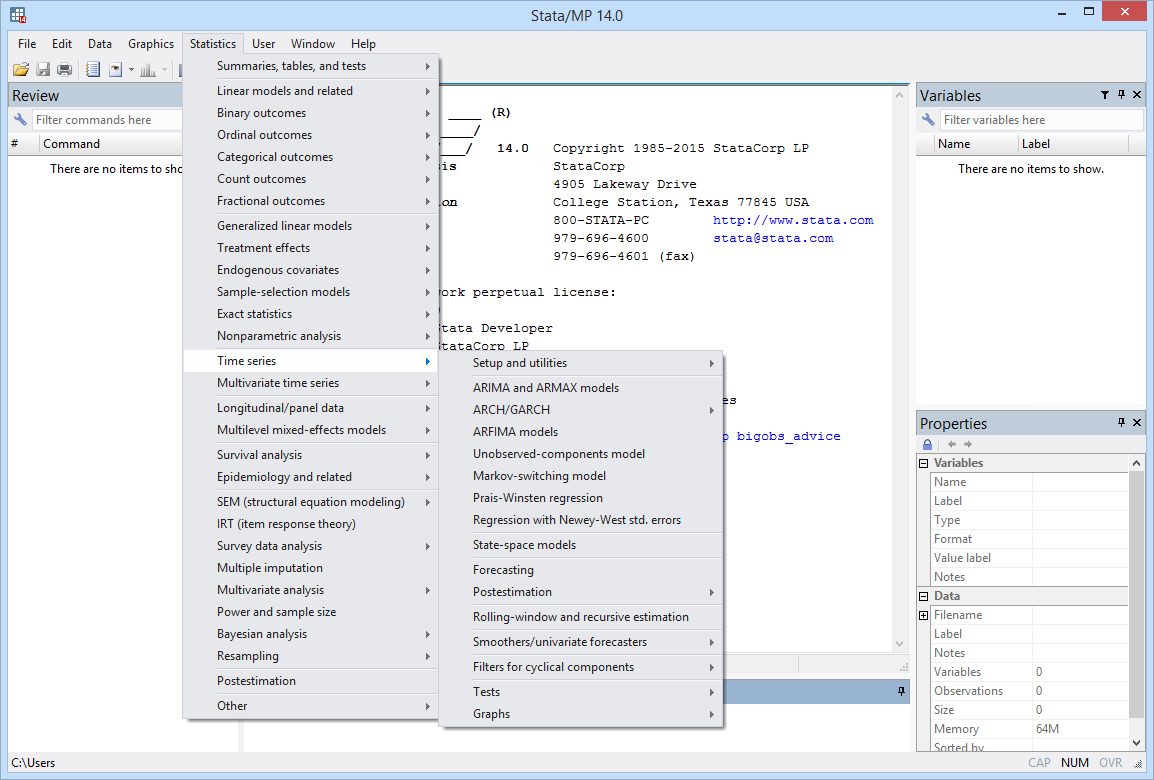ARIMA

ARMA

ARMAX

Standard and robust variance estimates

Static and dynamic forecasts

Linear constraints

Multiplicative seasonal ARIMA

Spectral densities

Impulse-response functions (IRFs)

Parametric autocorrelation estimates and graphs

Check stability conditions

ARCH/GARCH

GARCH

APARCH

EGARCH

NARCH

AARCH

GJR and more

ARCH in mean

Standard and robust variance estimates

Normal, Student’s t, or generalized error distribution

Multiplicative deterministic heteroskedasticity

Static and dynamic forecasts

Linear constraints

Multivariate GARCH

Diagonal VECH models

Conditional correlation models

Constant conditional correlation

Dynamic conditional correlation

Varying conditional correlation

Multivariate normal or multivariate Student’s t errors

Standard and robust variance estimates

Static and dynamic forecasts

Linear constraints

Markov-switching models

Dynamic regression

Autoregression

Tables of transition probabilities

Tables of expected durations

Standard and robust variance estimates

Watch Markov-switching models.

ARFIMA

Long-memory processes

Fractional integration

Standard and robust variance estimates

Static and dynamic forecasts

Linear constraints

Spectral densities

Impulse-response functions (IRFs)

Parametric autocorrelation estimates and graphs

Regression with AR(1) disturbances

Heteroskedasticity-and-autocorrelation-consistent covariance matrices

Cochrane–Orcutt/Prais–Winsten methods

ARMA/ARIMA estimators

ARCH estimators

Unobserved components model (UCM)

Trend-cycle decomposition

Stochastic cycles

Estimation by state-space methods

Standard and robust variance estimates

Static and dynamic forecasts

Linear constraints

Spectral densities

FRED data

Over 470,000 U.S. and international economic and financial time series

Search or browse by subject, title, or source

Put series on a common periodicity

Easily update datasets containing dozens, or even hundreds, of series

Easy-to-use interface for searching and browsing

Commands for updating datasets and replicability

Create calendar from dataset

Format variables using business calendar format

Convert between business dates and regular dates

Lags and leads calculated according to calendar

Graphs and tables

Autocorrelations and partial correlations

Cross-correlations

Cumulative sample spectral density

Periodograms

Line plots

Range plot with lines

Patterns of missing data

Watch Line graphs and tin().

Time-series functions

String conversion to date: daily, weekly, monthly, quarterly, half-yearly, yearly

Dates and times from numeric arguments

Date and time literal support

Periodicity conversion, e.g., daily date to quarterly

Date and time ranges

Time-series operators

L, lag

D, differences

S#, seasonal lag

Watch Time-series operators.

Time-series time and date formats

Default formats for clock-time daily, weekly, monthly, quarterly, half-yearly, yearly

High-frequency data with millisecond resolution

User-specified formats

Support for Haver Analytics database

New import haver command makes using Haver datasets even easier

Quickly access worldwide economics and financial datasetsForecast models

Combine results from multiple estimation commands

Specify identities and declare exogenous variables

Obtain dynamic and static forecasts

Use simulation methods to obtain prediction intervals

Specify alternative scenarios and perform “what-if” analyses

Watch A tour of forecasting.

VAR/SVAR/VECM

Vector autoregression (VAR)

Structural vector autoregression (SVAR)

Vector error-correction models (VECM)

Impulse–response functions (IRFs)

Simple IRFs

Orthogonalized IRFs

Structural IRFs

Cumulative IRFs

Dynamic multipliers

Forecast-error variance decompositions (FEVD)

Static and dynamic forecasts

Diagnostics and tests

Cointegration tests

Granger causality tests

LM tests for residual autocorrelation

Tests for normality of residuals

Lag-order selection statistics

Stability analysis using eigenvalues

Wald lag-exclusion statistics

Graphical and tabular presentations and comparisons of IRFs and FEVDs

State-space models

VARMA models

Structural time-series models

Stochastic general-equilibrium models

Stationary and nonstationary models

Standard and robust variance estimates

Static and dynamic forecasts

Linear constraints

Dynamic-factor models

Unobserved factors with vector autoregressive structure

Exogenous covariates

Autocorrelated disturbances in dependent variables’ equations

Standard and robust variance estimates

Static and dynamic forecasts

Linear constraints

Threshold regression

One threshold or multiple thresholds

Specify number of thresholds

Automatically choose the number of thresholds, using

BIC

AIC

Hannan-Quinn information criterion

Thresholds may be:

Points in time

Values of covariates in the regression model

Values of variables not in the regression model

Watch Threshold regression.

Postestimation Selector

View and run all postestimation features for your command

Automatically updated as estimation commands are run

Watch Postestimation Selector.

Tests for structural breaks

Unknown break point

Known break points

Cumulative sum test for stability of coefficients

Tests for white noise

Portmanteau’s test

Bartlett’s periodogram test

Regression diagnostics

LM test for ARCH effects

Breusch–Godfrey LM test for serial correlation

Durbin alternative test for serial correlation

Durbin–Watson statistic

Tests for unit roots

Dickey–Fuller

Modified Dickey–Fuller t test proposed by Elliott, Rothenberg, and Stock

Augmented Dickey–Fuller test

Phillips–Perron

Time-series filters

Baxter–King band-pass filter

Butterworth high-pass filter

Christiano–Fitzgerald band-pass filter

Hodrick–Prescott high-pass filter

Time-series smoothers

Moving average (MA)

Single exponential

Double exponential

Holt–Winters nonseasonal exponential

Holt–Winters seasonal exponential

Nonlinear

Forecasting and smoothing

Watch Moving-average smoothers.

Rolling and recursive estimation

Introduction to Time Series Using Stata by Sean Becketti

Financial Econometrics Using Stata by Simona Boffelli and Giovanni Urga

In the spotlight: State-space models: Easier than they look

In the spotlight: mgarch

In the spotlight: New univariate time-series features added in 13.1

In the spotlight: forecast for dynamic panel data and counterfactuals

NetCourse® 461: Introduction to Univariate Time Series with Stata